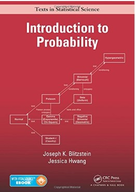Introduction To Probability - 1 Edition - Chapter 2 - Problem 22
Register Now

Join StudySoup

Get Full Access to Introduction To Probability - 1 Edition - Chapter 2 - Problem 229781466575578

# A bag contains one marble which is either green or blue, with equal probabilities. A

Introduction to Probability | 1st Edition

Problem 22

A bag contains one marble which is either green or blue, with equal probabilities. A green marble is put in the bag (so there are 2 marbles now), and then a random marble is taken out. The marble taken out is green. What is the probability that the remaining marble is also green?

Accepted Solution
Step-by-Step Solution:
Step 1 of 3

Stats 401 Week 2 Relative frequency = How many times appeared in category ÷ Total items in category (like finding percent without multiplying by 100 at the end) For the median, if there is an even amount of number given then add the two middle terms then divide by 2. EX: 1 2 3 4 5 6. The two middle terms are 3 and 4 so 3 + 4 = 7. Then 7/2 = 3.5 (finding the average of those numbers). If there isn’t a mode (1 2 3 4 5 6) then write “NO MODE” not “The mode is zero” because that implies that there is the number 0 and that is what appears the most. Peak of curve is in the middle When the mean = median = mode the distribution is bell shaped or symmetric. Median > mean then it is left skewed Med

###### Chapter 2, Problem 22 is Solved

Step 2 of 3

Step 3 of 3

Unlock Textbook Solution